Function Repository Resource:

# ProductD

Evaluate the product derivative of a function

Contributed by: Jan Mangaldan
 ResourceFunction["ProductD"][f,x] gives the product derivative of the function f with respect to x. ResourceFunction["ProductD"][f,{x,n}] gives the nth multiple product derivative of the function f with respect to x.

## Details

The product derivative is also known as the multiplicative derivative or geometric derivative.
The first product derivative ResourceFunction["ProductD"][f[x],x] is defined as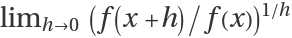.
The second product derivative ResourceFunction["ProductD"][f[x],{x,2}] is the product derivative of the first product derivative; higher-order product derivatives are defined analogously.
The order of derivative n should be a non-negative integer.

## Examples

### Basic Examples (2)

Product derivative of xa:

 In:=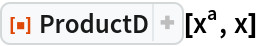Out=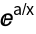Third product derivative of ex:

 In:=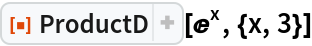Out=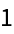### Scope (2)

Product derivative of a polynomial:

 In:=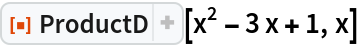Out=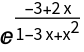Product derivative of the product logarithm:

 In:=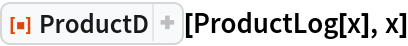Out=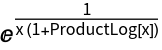### Properties and Relations (4)

The product derivative of a function is defined as a limit:

 In:=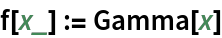In:=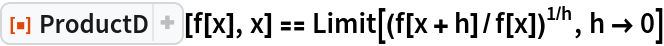Out=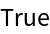Higher-order product derivatives can be defined recursively:

 In:=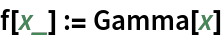In:=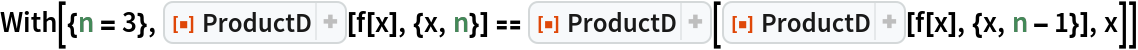Out=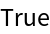In:=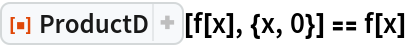Out=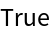Product rule for the product derivative:

 In:=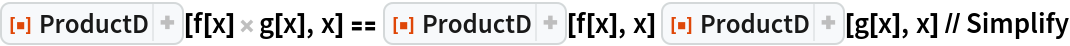Out=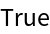Quotient rule for the product derivative:

 In:=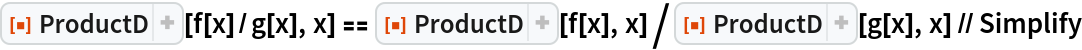Out=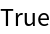### Neat Examples (1)

A function whose product derivative is itself:

 In:=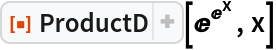Out=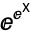## Version History

• 1.0.0 – 02 March 2021